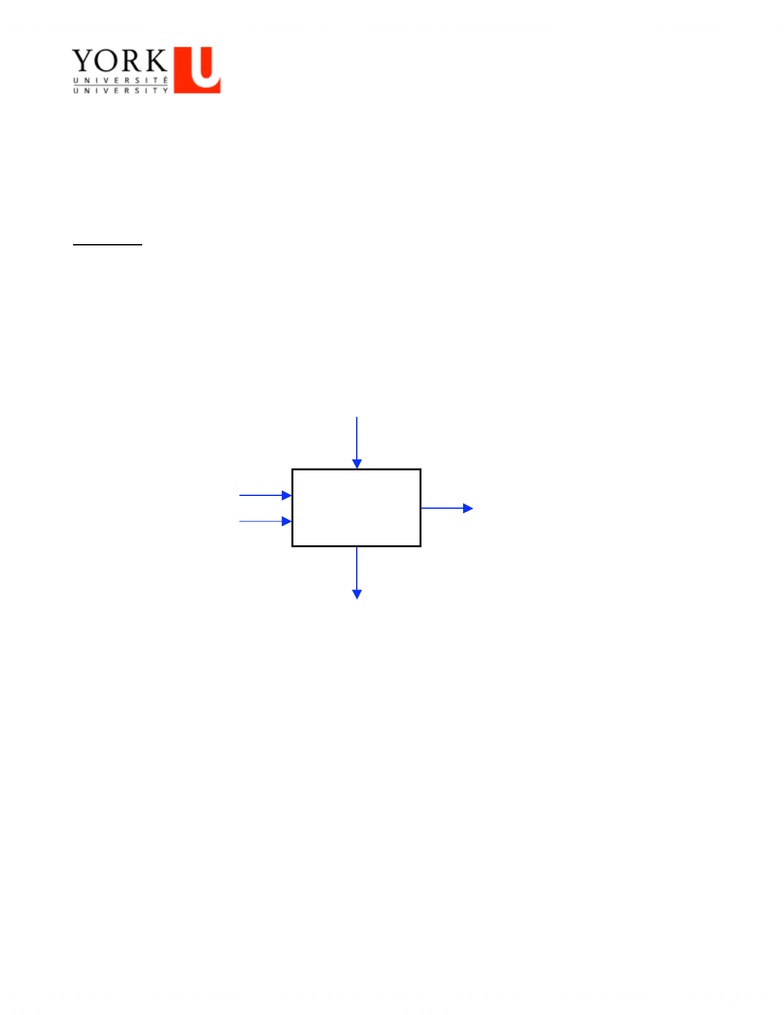# EECS 2021 Lecture Notes - Slacklining, Opcode, Register File

139 views3 pages
School
York University
Department
Electrical Engineering and Computer Science
Course
EECS 20211
CSE 2021: Computer Organization
Fall 2010
Assignment # 2: ALU Design and Datapath
Due Date: December 06, 2010
Please note that a short quiz based on Assignment 2 will be held in class on Monday, December 06, 2010 to
assess your performance. Students should complete the assignment accordingly. Good luck!
Question 1: (Digital Logic Design)
Instead of using 2's complement to handle subtraction, an unconventional machine uses a different approach. It
performs subtraction in the ALU by using a subtractor logic circuit obtained by cascading several 1-bit
subtractors. A 1-bit subtractor is explained below.
A full 1-bit subtractor has three 1-bit inputs (two data inputs and a borrow input from the previous stage) and
two 1-bit outputs (a difference bit and a borrow output bit to the next stage). A full subtractor is shown in fig.1
where the data inputs are represented by a and b. We perform the operation (a b).
Fig. 1: Schematic diagram for a 1-bit subtractor
Just as adding two numbers bit-by-bit generates carries, subtracting two numbers generates borrows that ripple
from one bit position to the next. A 1 borrowed by the previous bit is shown in fig. 1 as the borrow-in bit (bin)
and is an input to the subtractor. If needed, a 1 is borrowed from the next bit location to perform the subtraction
(ab) and is an output, shown as borrow-out (bout) in fig. 1. For the 1-bit subtractor illustrated in fig. 1:
a) Draw the truth table with three inputs (a, b, and bin) and two outputs (bout and D).
b) Based on the truth table sketched in a), derive a Boolean expression in sum-of-product representation for
each of the outputs (bout and D).
c) Using AND, OR, and NOT gates, draw digital circuits that implement the outputs (bout and D) from the
inputs (a, b, and bin).
d) Using the schematic shown in fig. 1, implement a 32-bit subtractor.
+
1-bit
Subtractor
a
b
Borrowin
(bin)
Difference
(D)
Borrowout
(bout)
Unlock document

This preview shows page 1 of the document.
Unlock all 3 pages and 3 million more documents.

## Document Summary

Please note that a short quiz based on assignment 2 will be held in class on monday, december 06, 2010 to assess your performance. Instead of using 2"s complement to handle subtraction, an unconventional machine uses a different approach. It performs subtraction in the alu by using a subtractor logic circuit obtained by cascading several 1-bit subtractors. A full 1-bit subtractor has three 1-bit inputs (two data inputs and a borrow input from the previous stage) and two 1-bit outputs (a difference bit and a borrow output bit to the next stage). A full subtractor is shown in fig. 1 where the data inputs are represented by a and b. Just as adding two numbers bit-by-bit generates carries, subtracting two numbers generates borrows that ripple from one bit position to the next. A 1 borrowed by the previous bit is shown in fig. 1 as the borrow-in bit (bin) and is an input to the subtractor.